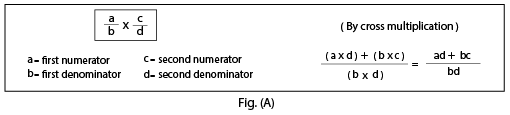Sum of fractions with different denominators

## Objective

To find the difference of two unlike fraction.

## Pre-requisite Knowledge

• Concept of Addition of natural numbers.
• Concept of fractions.

## What is Fraction?

A fraction is a numerical value that represents the parts of a whole.

## Parts of a Fraction

A fraction consists of two parts, namely the numerator and the denominator. The upper part of the fraction is called the numerator, and the lower part of the fraction is called the denominator. For example, 4/7 is a fraction. Here, 4 is the numerator and 7 is the denominator.

## Unlike fraction

Fractions with the different denominators are called unlike fractions.

Thus, 1/5, 2/7, 3/2, 8/5 are all unlike fractions.Addition of unlike fractions means add one fraction to the second fraction.